Physics Paper 2, May/June 2012
 Questions: 1 2 3 4 5 6 7 8 9 10 11 12 13 14 15 Main
Weakness/Remedies
Strength

Question 13

1. Explain the term critical angle
2. list two factors which determine the deviation of a ray of light by a triangular glass prism
3. The angle of refraction (r) of a ray of white light from air through a triangular glass prism of refractive index 1.5 is 29.0°. Calculate the angle through which the ray it least deviated.
4. Study the ray diagram below and use it to answer the questions that follow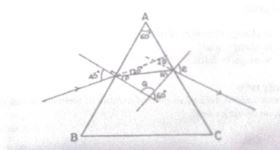Calculate the:

1. Values of angles P,Q and R;
2.   Refractive index n of the glass prism;
3. value of e;
4. Total deviation D
_____________________________________________________________________________________________________

Part (a) Majority of the candidates merely defined critical angle without adding the necessary additional information so they lost some marks. Performance was above average

Part (b) majority of the candidates had no difficulty in answering this question.

Part (c) most candidates assumed the angle of the prism to be 60°. this is not correct. They are expected to ca;culate it out from the given information in the question i.e
A= 2r = 2 x 29 = 58° . Few candidates did this and scored full marks

Part (d) (i) and (ii) posed no problem to majority of the responding candidates. However in (iii)
and (IV) candidates again assumed the problem to be minimum deviation problem which is not
correct. Performance was average.                                                       -
(a) Critical angle is the angle of incidencc in the denser medium when the angle of refraction in the less dense medium is 90°
e.g correct diagram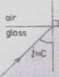(b) Factors affecting the deviation of a rav in a triangular glass prism
angle of incidence
refracting angle of the prism
refractive index of the material of the prism

(C)   n =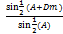A = 2r
= 2 x 29
= 58
Sin ½ (A+D) = n Sin ½ A
½ (A+Dm) =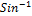(1.5 x Sin½ x 58)
=0.7272 =  46.67
58 + Dm = 93.32
Dm= 35.32

(d)  (i) Angle P = 45-20
=  25°
Angle Q = 180 – 60
=   120°
P + Q + R = 180
R = 180 - (P +Q)
= 180 – 145
= 35°

1. n =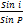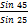=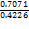= 1.673

1. n =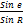1.673 =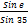Sin e = 1.673 x Sin 35
= 0.9595
E = sin-1(0.9595)
=73.7°

1. D = (i+e) – A

= (45 + 73.3) – 60
= 58.3°